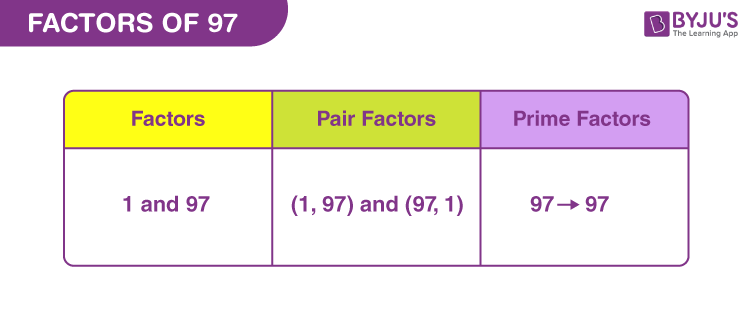# Factors of 97

Factors of 97 are the whole numbers that can divide the original number, completely. When the product of two integers results in 97, then they are the respective factors.  97 is an odd number, thus we cannot divide it by 2.

If ‘x’ is the factor of 97, then ‘x’ divides 97 into equal parts. For example, if 4 is the factor of 16, then 16 divided by 4 is equal to 4. Thus, 4 divides 16 into four equal parts.

16 ÷ 4 = 4 [Remainder = 0 and quotient = 3]

Let us find the factors, pair factors and prime factors of 97 in this article with simple methods.## How to Find the Factors of 97?

Factors are the real numbers that can divide the actual number, evenly. By the list of the prime numbers, we know, 97 is the 25th prime number. Therefore, 97 will have only two factors, 1 and 97. We cannot find any other factor of ninety-seven.

97 ÷ 1 = 97

97 ÷ 97 = 1

If we divide 97 by any other integer, then the quotient produced after division will be a fraction or decimal number. Thus, the factors of 97 are 1 and 97.

## Pair Factors of 97

The multiplication of pair factors of 97 will result in the actual number. Hence,

1 × 97 = 97

Or

97 × 1 = 97

Therefore, there is only one pair factor, i.e. (1, 97).

Similarly, we can also consider negative pair factors that will result in the original number.

-1 × -97 = 97

Therefore, the negative pair factor is (-1, -97).

## Prime Factorisation of 97

By the prime factorization method, we can easily find the prime numbers that are the factors of the original number. As we already know 97 is a prime number, therefore, the prime factorization of 97 is not required.

Check:

We can check if 97 is a prime number or not, by dividing it by other prime numbers. First let us list down the prime numbers from 1 to 100, excluding 97.

 2, 3, 5, 7, 11, 13, 17, 19, 23, 29, 31, 37, 41, 43, 47, 53, 59, 61, 67, 71, 73, 79, 83, 89

Now if we start dividing 97 by 2, then we get;

97/2 = 48.5

97/3 = 32.33

97/5 = 19.4

97/7 = 13.85

Continue dividing up to 89, we will see all the resultant quotients are fractions. Therefore, we can conclude that 97 is a prime number.

Therefore,

 Prime factorisation of 97 = 97

## Video Lesson on Prime Factors## Solved Examples

Q.1: Find the sum of all the factors of ninety-seven.

Solution: The factors of 97 are 1 and 97.

Sum = 1 + 97 = 98

Therefore, 98 is the required sum.

Q.3: What is the greatest common factor of 92, 93, 95 and 97?

Answer: Let us list down the factors of all the numbers.

Factors of 92 → 1, 2, 4, 23, 46, 92

Factors of 93 → 1, 3, 31, 93

Factors of 95 → 1, 5, 19, 95

Factors of 97 → 1, 97

Hence, we can see, 1 is the only factor that is common to all. So,

GCF (92, 93, 95, 97) = 1

## Frequently Asked Questions on Factors of 97

### 1. What are the factors of 97?

Answer: There are only two factors of 97, they are 1 and 97, itself.

### 2. What are the multiples of 97?

Answer: The first ten multiples of 97 are 97, 194, 291, 388, 485, 582, 679, 776, 873 and 970.

### 3. Is 97 a prime or composite number?

Answer: 97 is a prime number.

### 4. How many positive factors do 97 have?

Answer: 97 has only two positive factors, 1 and 97.

### 5. Is 97 divisible by any other number?

Answer: 97 is not divisible by any other real number, apart from 1 and the number itself.

### 6. Is 97 a perfect square?

Answer: 97 is not a perfect square, because the root of 97 is not a whole number but a fraction.